### Using SET Operators in SQL Server

In this article, we will see how to use SET Operators in SQL Server. We will look at UNION, UNION ALL, INTERSECT and EXCEPT operators.

To start with SET Operators, we will first create two sample tables in our Northwind database. The script is shown as below -

USE Northwind
GO

CREATE TABLE Example1
(
Name CHAR(1)
)

CREATE TABLE Example2
(
Name CHAR(1)
)

--INSERT FOR Example1 Table
INSERT INTO Example1 VALUES('A')
INSERT INTO Example1 VALUES('B')
INSERT INTO Example1 VALUES('A')
INSERT INTO Example1 VALUES('C')
INSERT INTO Example1 VALUES('D')
INSERT INTO Example1 VALUES('E')

--INSERT FOR Example2 Table
INSERT INTO Example2 VALUES('X')
INSERT INTO Example2 VALUES('A')
INSERT INTO Example2 VALUES('B')
INSERT INTO Example2 VALUES('B')
INSERT INTO Example2 VALUES('C')
INSERT INTO Example2 VALUES('Z')
INSERT INTO Example2 VALUES('Y')

--SELECT ROWS FROM BOTH THE TABLES [Example1, Example2]
SELECT * FROM Example1
SELECT * FROM Example2

In the above script, we have created two tables, Example1 and Example2 and inserted some dummy data. We will now take a look at how to select the data from both the tables using the SET Operators.

## UNION Operator

Let’s take an overview of our very first operator - UNION. The UNION operator combines the result of two or more queries and returns a single result set excluding the duplicate values. The following figure shows the same output -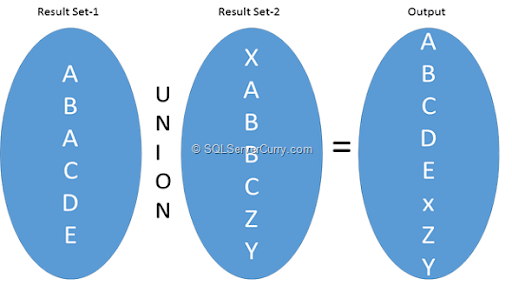Write the following query in SQL Server Management Studio and observe the result -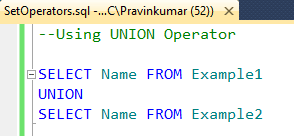The output of the above query is as shown here -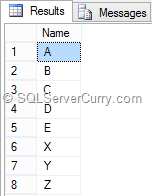Now let’s use the two tables in the Northwind database with the name Dept and Emp.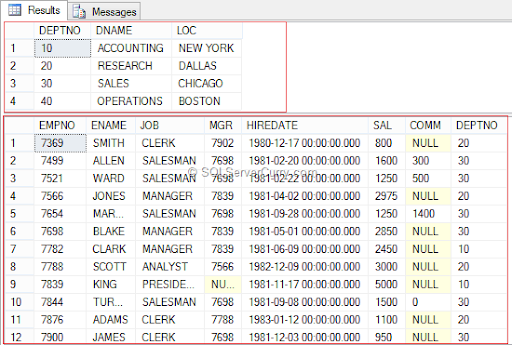We will write a query where we want to find all the unique cities and countries from Customers and Employees table -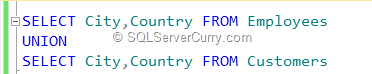The output is shown below -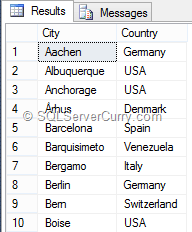## UNIONALL Operator

However if you want to include the duplicate values of City and Country from Customers, as well as Employees, include ALL with UNION. The query is shown below -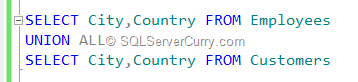Some rules to remember while working with UNION operator -

1. The number of columns must be same.
2. The data type of the columns must be same or implicitly convertible by database.

## INTERSECT Operator

We will now take a look at INTERSECT operator. The INTERSECT operator takes the result of two queries and returns common rows which appears in both the result sets excluding the duplicate values. The following figure explains the same -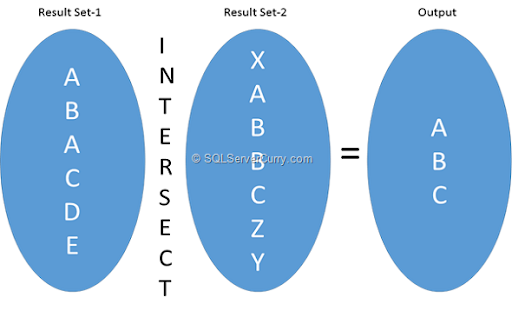Let's try the query on our Example1 and Example2 table. The query is shown below -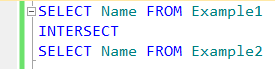The output of the above INTERSECT query is shown below -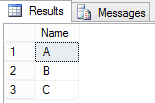We will try the INTERSECT on Northwind database and find out all the common Cities and countries from Customers and Employees tables excluding duplicates. The query is as shown below -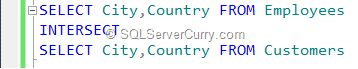The output of the above query is as shown below -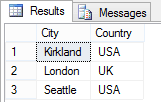## EXCEPT Operator

We will now explore the last operator, the EXCEPT operator. The EXCEPT operator returns distinct rows from the first query which do not appear into the second result set. The following figure explains the same -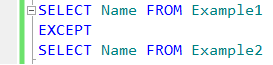The output of the above query is shown below -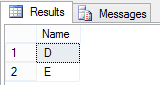We will now try the same query with our Northwind database. We will find out all the distinct cities from Employees tables which does not appear in Customers table. The query is shown below -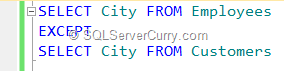The output of the above query is shown below -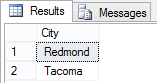And that’s it. In this article, we have seen how to use SET Operators in SQL Server. We have seen UNION, UNION ALL, INTERSECT and EXCEPT operator with some simple examples.